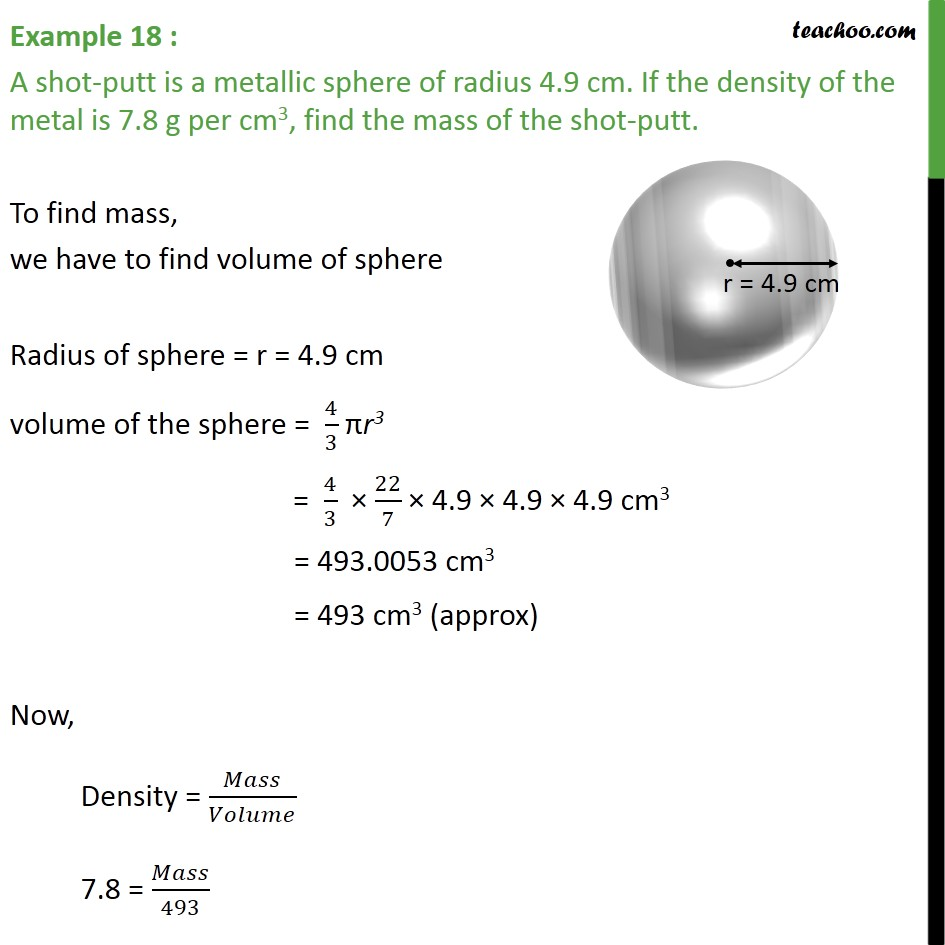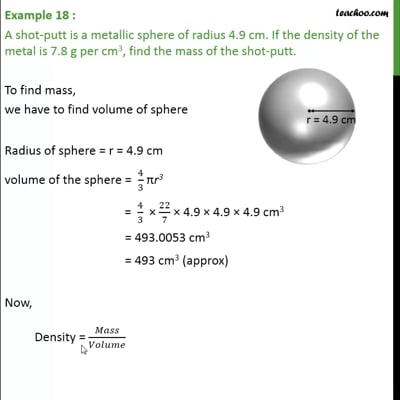Examples

Chapter 13 Class 9 Surface Areas and Volumes (Term 2)
Serial order wiseThis video is only available for Teachoo black users

### Transcript

Example 18 : A shot-putt is a metallic sphere of radius 4.9 cm. If the density of the metal is 7.8 g per cm3, find the mass of the shot-putt. To find mass, we have to find volume of sphere Radius of sphere = r = 4.9 cm volume of the sphere = 4/3 r3 = 4/3 22/7 4.9 4.9 4.9 cm3 = 493.0053 cm3 = 493 cm3 (approx) Now, Density = / 7.8 = /493 /493 = 7.8 Mass = 7.8 493 g Mass = 3845.44 g